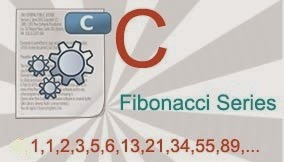# Fibonacci Series in CFibonacci series a commonly asked question in school or university examinations and interviews! Today we will  learn and discuss the logic to generate Fibonacci series in C programming, Let's Start!

## What is Fibonacci Series?

Fibonacci Sequence is the series of numbers starting from 0,1 and then rest sequence is generated by adding the previous two numbers and the same process continues for generating the rest series.
Fibonacci Sequence: 0, 1, 1, 2, 3, 5, 8, 13, 21, .....
So basically there's just one basic rule to remember is i.e, xn = xn-1 + xn-2

## Fibonacci Series in C

### Logic for Fibonacci Series

Take two variables n1 and n2 with initial value 0 and 1 respectively and a variable count / num which is used to track the number of terms and a check for our condition for Fibonacci series. After that print n1 and n2 and start a loop (using while in below codes) with the condition accordingly. In loop statement we have to assign new values to n1 and n2 and print the sum of n1 and nu before it. Let's understand this properly from the below source codes!

Fibonacci Series can be generated if we know:
1. Number of terms
2. Last term(number) condition
Note : Here we are taking input from user.

### Fibonacci Series in C: given number of terms

If we know the number of terms 'n' till which the Fibonacci series should be generated than, Fibonacci series will be generated using below source code.
```/* Fibonacci Series in C | for n terms */

#include<stdio.h>
#include<conio.h>

void main() {
int count,      //count num. of terms
n,          //max. num. of term in series
n1=0, n2=1;
printf(" Enter Number Of Terms: ");
scanf("%d",&n);
printf(" Fibonacci Series: %d %d ", n1, n2); //
count=2;    //since we printed 2 numbers 0 and 1
while (count<n) {
printf("%d ",n1+n2);
n2 = n1+n2 -n2 + (n1=n2); //assigning new values to n1 and n2
++count;
}
getch();
}```

Output:### Fibonacci Series in C: given last term condition

Similarly if we know the condition for the last term or number, than also Fibonacci series can be generated using below source code. Here we take a number input from user and the condition is, our series terms should less that that number. ex.- If use enter 5 than our series will be: 0 1 1 2 3  . Let's see how to do this!
```/* Fibonacci Series in C | for last term condition */

#include<stdio.h>
#include<conio.h>

void main() {
int num,      //last term <= num
n1=0, n2=1;
printf(" Enter a Number: ");
scanf("%d",&num);
printf(" Fibonacci Series: %d %d ", n1, n2); //
while (n1+n2 < num) {
printf("%d ",n1+n2);
n2 = n1+n2 -n2 + (n1=n2); //assigning new values to n1 and n2
}
getch();
}```

Output:## Fibonacci Series in C by Recursion

When a function call itself than the function is known as Recursive Function. Programs using Recursion generally have small  code and faster processing. So let's create Fibonacci Series program using recursion.
```/* Fibonacci Series in C Using Recursion */

#include<stdio.h>
#include<conio.h>

int fibonacci(int);

void main(){
int n, i = 0, count;
printf("Enter Number Of Terms: ");
scanf("%d",&n);
printf("Fibonacci series: ");

for (count=1; count<=n; count++, i++){
printf("%d ", fibonacci(i));
}

getch();
}

int fibonacci(int n) {
if (n == 0)
return 0;
else if (n == 1)
return 1;
else
return (fibonacci(n-1) + fibonacci(n-2));
}```

Conclusion:
Fibonacci series as said earlier is most simple and commonly asked question in C Programming. There are various other ways to generate the same Fibonacci sequence by using other logic and loop (for and do-while). In the above program we use a complex one line logic trick to swap two variables value while assigning new values to n1 and n2, a much understanding method can be done using a third variable and apply operations on them! Finally if you have any question regarding this program or any problem in programming... Kindly post your comment below